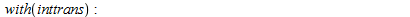# Maple question: defining functions as inverse Fourier transforms

• Maple
Homework Helper
Hi,

I have a a Fourier transformed variable $\hat{\eta}(k)$ defined as the following:

$$\hat{\eta}(k)=\frac{e^{-k^{2}}\tanh k}{kU^{2}+(-B+\Omega U+E_{b}|k|-k^{2})\tanh k}$$

The parameters $U,B,\Omega,E_{b}$ have all been defined previously. I have naively tried the following:

$$\eta :=\textrm{invfourier}(ETA,k,x)$$

This doesn't work. What am I doing wrong?

Cheers

Thanks for the post! This is an automated courtesy bump. Sorry you aren't generating responses at the moment. Do you have any further information, come to any new conclusions or is it possible to reword the post?

MathematicalPhysicist
Gold Member
First thing what is ETA?, you should write ##\hat{\eta}## in this place.
Second, if you read the help in maple then you should add at the beginning of the code: ""
I assume there's no easy term for the inverse fourier transform of this expression, but you can get an implicit expression with integral by adding in the last place the syntax: "NO_INT".

I also assume that you read already the help so I don't really no where you get it wrong, perhaps show us your code?

BTW, I find mapleprimes.com as the best place for my questions regarding maple (I am not advertising it's just my experience).